AP State Board Syllabus AP SSC 10th Class Maths Textbook Solutions Chapter 6 Progressions Ex 6.4 Textbook Questions and Answers.

## AP State Syllabus SSC 10th Class Maths Solutions 6th Lesson Progressions Exercise 6.4

### 10th Class Maths 6th Lesson Progressions Ex 6.4 Textbook Questions and Answers

Question 1.
In which of the following situations, does the list of numbers involved in the form a G.P.?
i) Salary of Sharmila, when her salary is Rs. 5,00,000 for the first year and expected to receive yearly increase of 10% .
Given: Sharmila’s yearly salary = Rs. 5,00,000.
Rate of annual increment = 10 %.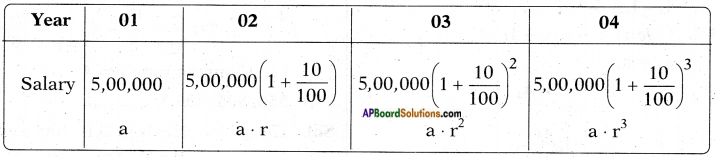Here, a = a1 = 5,00,000
a2 = 5,00,000 × $$\frac{11}{10}$$ = 5,50,000
a3 = 5,00,000 × $$\frac{11}{10}$$ × $$\frac{11}{10}$$ = 6,05,000
a4 = 5,00,000 × $$\frac{11}{10}$$ × $$\frac{11}{10}$$ × $$\frac{11}{10}$$ = 6,65,000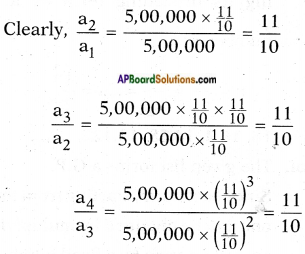Every term starting from the second can be obtained by multiplying its pre¬ceding term by a fixed number $$\frac{11}{10}$$.
∴ r = common ratio = $$\frac{11}{10}$$
Hence the situation forms a G.P.ii) Number of bricks needed to make each step, if the stair case has total 30 steps. Bottom step needs 100 bricks and each successive step needs 2 bricks less than the previous step.
Given: Bricks needed for the bottom step = 100.
Each successive step needs 2 bricks less than the previous step.
∴ Second step from the bottom needs = 100 – 2 = 98 bricks.
Third step from the bottom needs = 98 – 2 = 96 bricks.
Fourth step from the bottom needs = 96 – 2 = 94 bricks.
Here the numbers are 100, 98, 96, 94, ….
Clearly this is an A.P. but not G.P.

iii) Perimeter of the each triangle, when the mid-points of sides of an equilateral triangle whose side is 24 cm are joined to form another triangle, whose mid-points in turn are joined to form still another triangle and the process continues indefinitely.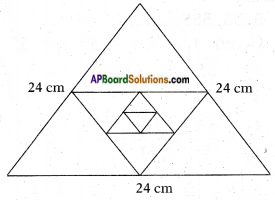Given: An equilateral triangle whose perimeter = 24 cm.
Side of the equilateral triangle = $$\frac{24}{3}$$ = 8 cm.
[∵ All sides of equilateral are equal] ……. (1)
Now each side of the triangle formed by joining the mid-points of the above triangle in step (1) = $$\frac{8}{2}$$ = 4 cm
[∵ A line joining the mid-points of any two sides of a triangle is equal to half the third side.]
Similarly, the side of third triangle = $$\frac{4}{2}$$ = 2 cm
∴ The sides of the triangles so formed are 8 cm, 4 cm, 2 cm,
a = 8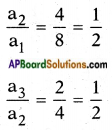Thus each term starting from the second; can be obtained by multiplying its preceding term by a fixed number $$\frac{1}{2}$$.
∴ The situation forms a G.P.

Question 2.
Write three terms of the G.P. when the first term ‘a’ and the common ratio ‘r’ are given.
i) a = 4 ; r = 3.
The terms are a, ar, ar2, ar3, ……..
∴ 4, 4 × 3, 4 × 32 , 4 × 32 , ……
⇒ 4, 12, 36, 108, ……

ii) a = √5 ; r = $$\frac{1}{5}$$
The terms are a, ar, ar2, ar3, ……..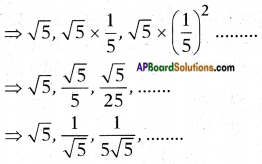iii) a = 81 ; r = –$$\frac{1}{3}$$
The terms of a G.P are:
a, ar, ar2, ar3, ……..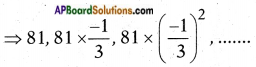⇒ 81, -27, 9,

iv) a = $$\frac{1}{64}$$; r = 2.
Given: a = $$\frac{1}{64}$$; r = 2.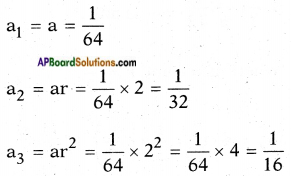∴ The G.P is $$\frac{1}{64}$$, $$\frac{1}{32}$$, $$\frac{1}{16}$$, …….

Question 3.
Which of the following are G.P. ? If they are G.P, write three more terms,
i) 4, 8, 16, ……
Given: 4, 8, 16, ……
where, a1 = 4; a2 = 8; a3 = 16, ……
$$\frac{a_{2}}{a_{1}}=\frac{8}{4}=2$$
$$\frac{a_{3}}{a_{2}}=\frac{16}{8}=2$$
∴ r = $$\frac{a_{2}}{a_{1}}=\frac{a_{3}}{a_{2}}$$ = 2
Hence 4, 8, 16, … is a G.P.
where a = 4 and r = 2
a4 = a . r3 = 4 × 23 = 4 × 8 = 32
a5 = a . r4 = 4 × 24 = 4 × 16 = 64
a6 = a . r5 = 4 × 25 = 4 × 32 = 128

ii) $$\frac{1}{3}$$, –$$\frac{1}{6}$$, $$\frac{1}{12}$$, …….
Given: t1 = $$\frac{1}{3}$$, t2 = –$$\frac{1}{6}$$, t3 = $$\frac{1}{12}$$, ….
$$\frac{1}{3}$$, –$$\frac{1}{6}$$, $$\frac{1}{12}$$, …….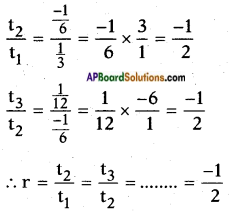Hence the ratio is common between any two successive terms.
∴ $$\frac{1}{3}$$, –$$\frac{1}{6}$$, $$\frac{1}{12}$$, ……. is G.P.
where a = $$\frac{1}{3}$$ and r = –$$\frac{1}{2}$$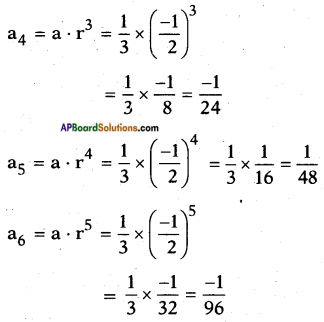iii) 5, 55, 555, ……..
Given: t1 = 5, t2 = 55, t3 = 555, ….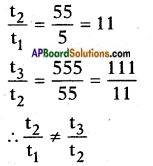∴ 5, 55, 555, …….. is not a G.P.

iv) -2, -6, -18, ……
Given: t1 = -2, t2 = -6, t3 = -18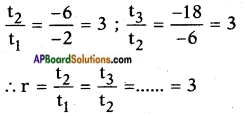∴ -2, -6, -18, is a G.P.
where a = -2 and r = 3
an = a . rn-1 =
a4 = a . r3 = (-2) × 33 = -2 × 27 = -54
a5 = a . r4 = (-2) × 34 = -2 × 81 = -162
a6 = a . r5 = (-2) × 35 = -2 × 243 = -486

v) $$\frac{1}{2}$$, $$\frac{1}{4}$$, $$\frac{1}{6}$$, …….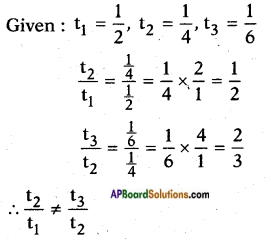i.e., $$\frac{1}{2}$$, $$\frac{1}{4}$$, $$\frac{1}{6}$$, ….. is not a G.P.

vi) 3, -32, 33, ……
Given: t1 = 3, t2 = -32, t3 = 33, ……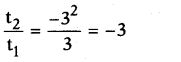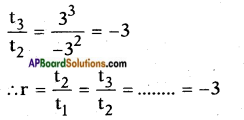i.e., every term is obtained by multiplying its preceding term by a fixed number -3.
3, -32, 33, …… forms a G.P,
where a = 3; r = -3
an = a . rn-1
a4 = a . r3 = 3 × (-3)3 = 3 × (-27) = -81
a5 = a . r4 = 3 × (-3)4 = 3 × 81 = 243
a6 = a . r5 = 3 × (-3)5 = 3 × (-243) = -729

vii) x, 1, $$\frac{1}{x}$$, …….
Given: t1 = x, t2 = 1, t3 = $$\frac{1}{x}$$, ……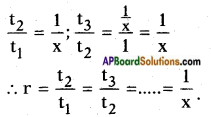Hence x, 1, $$\frac{1}{x}$$, …. forms a G.P.
where a = x; r = $$\frac{1}{x}$$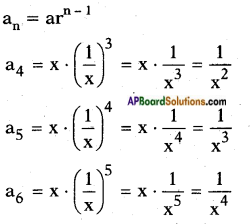viii) $$\frac{1}{\sqrt{2}}$$, -2, $$\frac{8}{\sqrt{2}}$$, …….
Given: t1 = $$\frac{1}{\sqrt{2}}$$, t2 = -2, t3 = $$\frac{8}{\sqrt{2}}$$, ……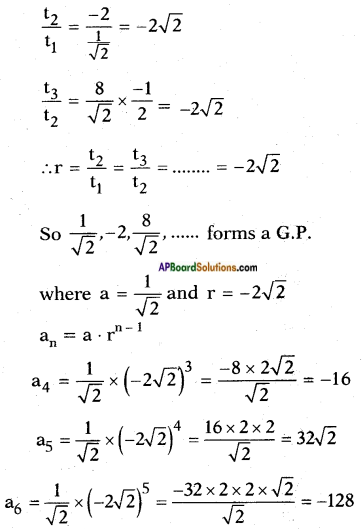ix) 0.4, 0.04, 0.004, ……..
Given: t1 = 0.4, t2 = 0.04, t3 = 0.004, ……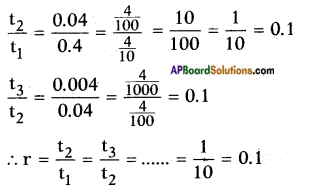∴ 0.4, 0.04, 0.004, …….. forms a G.P.
where a = 0.4; r = $$\frac{1}{10}$$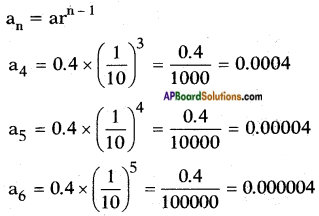Question 4.
Find x so that x, x + 2, x + 6 are consecutive terms of a geometric progression.
∴ r = $$\frac{\mathrm{t}_{2}}{\mathrm{t}_{1}}$$ = $$\frac{\mathrm{t}_{3}}{\mathrm{t}_{2}}$$
⇒ $$\frac{x+2}{x}$$ = $$\frac{x+6}{x+2}$$